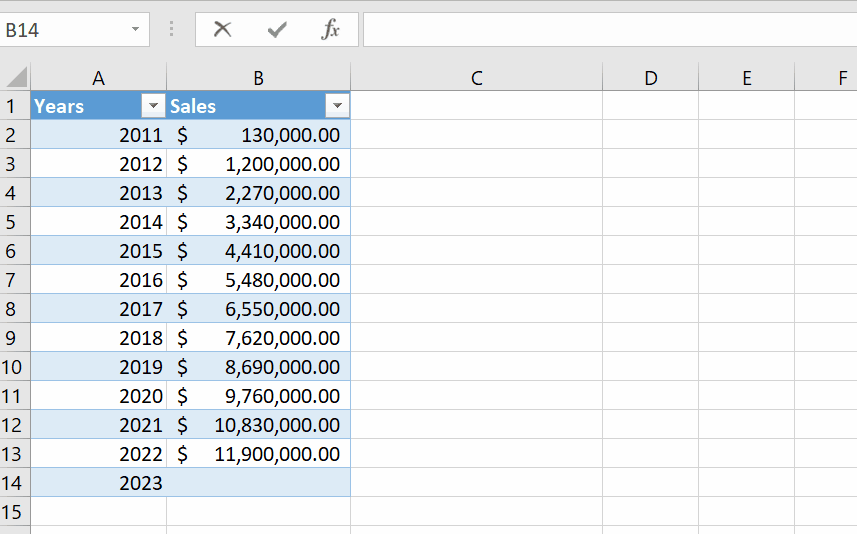# How calculate growth in Excel

In this tutorial we will learn how to find growth in Excel by using the growth formula. The exponential GROWTH function in Excel is a statistical function that returns the predictive exponential growth for a given set of data. A given new value of x returns the predicted value of y. In addition, the Growth formula in Excel helps in financial and statistical analysis.

For example , If you have data of any company or business. The sales at x-axis and the year passed at y-axis we can easily find the growth by using the built -in function of Microsoft Excel the growth formula.

The Formula is

=Growth(known_y’s , known_x’s , new_x’s ,[const])

For the GROWTH formula in Excel, y =b* m^x represents an exponential curve where the value of y depends upon the value x, m is the base with exponent x, and b is a constant value.

For a given relation, y =b*m^x

Known_y’s: It is a set of y-values in the data set. It is a required argument.

Known_x’s: It is a set of x-values in the data set. The range value for known_x’s should be the same as known_y’s values. It is an optional argument. If known_x’s is omitted, it is assumed to be the array (1,2,3,…) the same size as known_y’s.

New_x’s: t is the new value of x for which we want to calculate the corresponding predictive value. If we omit this value, the new value of x the function that the value as the same value of known x’s and based on that value, it returns the value of y. New_x’s must include a column (or row) for each independent variable, just as known_x’s.

Const: It is also an optional argument that tells whether the constant b for equation y= b* m^x is equal to 1. It is whether the constant value is true or omitted. The value of b is calculated normally. Else if the constant value is false, the value of b is set equal to 1, and the values of m are adjusted such that we have the relation y = m^x.

Follow the step below to find  growth for next year from a company’s sales data.Microsoft Excel is famous around the globe due to its fast calculation and numeric data manipulation by built-in tools and functions. It is also used for forecasting of a business in different dimensions. Microsoft Excel has a built-in function to calculate the growth of any business.

### Step 1 – Select the cell and apply the formula– Select any cell where you wish to calculate growth.
– Type the formula =GROWTH(B2:B13,A2:A13,A14).
– As soon as you press the enter key it will show the expected sales of 2023.

Hence, we have found the expected sales / growth of 2023 year by above shown animation.